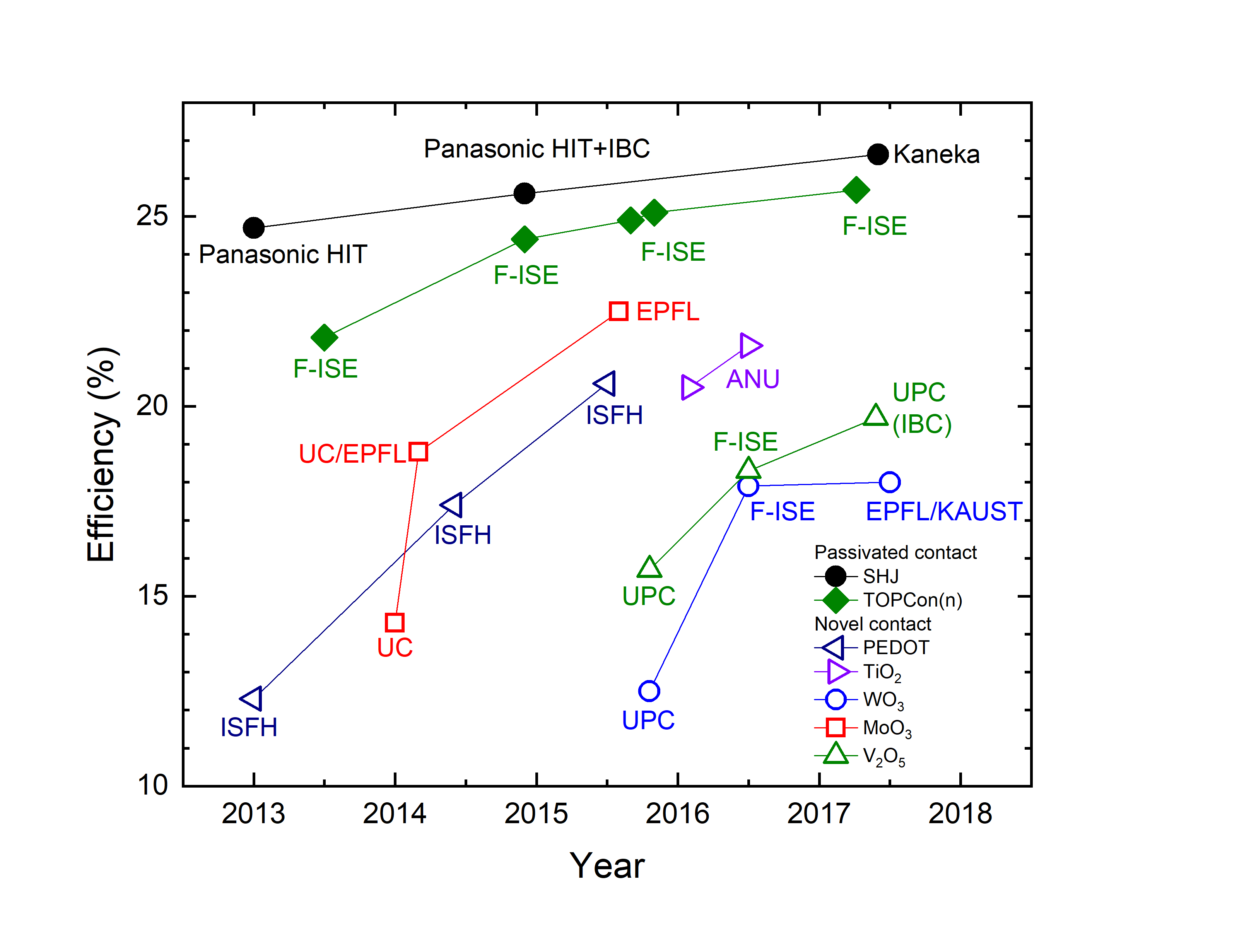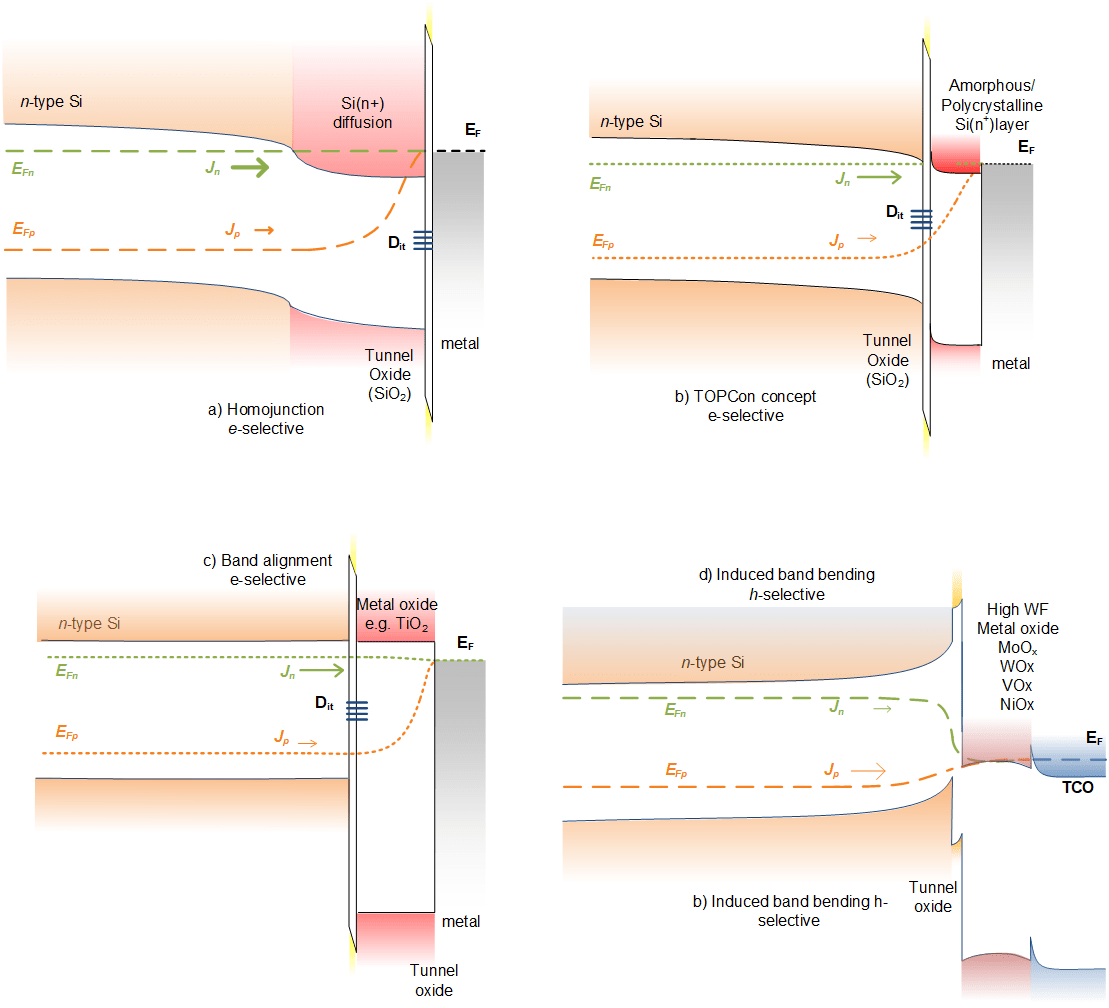# Carrier selective contacts for silicon solar cells

It is clear that to further improve the efficiency it is necessary to reduce the electrical losses at the surfaces and specifically the contact between the semiconductor and the metallisation. Current technology has pushed this to their limit and new disruptive solutions are now required. As a result carrier selective or passivating contacts have recently attracted significant interest in the photovoltaic scientific community . These passivating or carrier-selective contacts by design exhibit extremely low recombination currents for minority carriers (i.e. passivation) while having a low resistivity for majority carriers (i.e., carrier selectivity). An elegant way to look at this was recently proposed by Brendel et al.  Based on the theoretical framework proposed by Wuerfel et al.  which attributes carrier selectivity to the asymmetry in the electron and hole conduction, Brendel et al. defined the electron resistance ρn and hole resistance ρp as follows (in this case for a hole-selective contact):$\rho_n=(\frac{dJ_n}{\partial dV})^{-1}=\frac{v_{th}}{f_c j_c }$  (1)$\rho_p=(\frac{dJ_p}{dV})^{-1}=\frac{\rho_c}{f_c}$  (2)

Where Jn,p is the electron/hole current, V is the voltage, vth is the thermal voltage, fc is the contact fraction, and jc is the minority carrier combination current (both at V=0 V. When dividing the electron and hole resistance we can define the selectivity S10:$S_{10}=log_{10}( \frac{\rho_n}{\rho_p})=log_{10} (\frac{v_{th}}{j_c \rho_c})$  (3)

where log10 is the logarithm with base 10. This selectivity is a very useful parameter to consider as it is an excellent figure of merit to estimate the efficiency potential of a certain contact. This is shown schematically in Figure 1 where we plot the upper limit efficiency as a function of the jc and ρc of a contact. It should be noted that you can move along the iso-selectivity lines (dotted lines in Figure 1) by changing the contact fraction of the contact. This is for example already exploited in the PERC solar cell where the optimal rear contact fraction (with a selectivity of 13 ) is around 1% thereby limiting the solar cell efficiency to 27.2%. Unfortunately, the selectivity of the electron contact in a PERC cell is ~12  and this limits the PERC solar cell to roughly 25% in very good agreement with champion results achieved by UNSW in 1999 .Figure 1 (a): Upper limit efficiency of a silicon solar cell as a function of the minority carrier recombination current jc and majority carrier contact resistance ρc. The dashed lines indicate iso-selectivity lines. Please note that according to the definitions in Eq. 1-3, we can move along the iso-selectivity line by changing the contact fraction fc. Hence, this allows for optimising the efficiency ceiling for a certain contact system when the jc and ρc  values are known. This calculation was adapted from Brendel et al.  and assumes a short-circuit density of 43.6 mA/cm2 and assumes that the solar cell efficiency is limited by only one contact.Figure 1 (b) Shows the campion efficiencies of the various carrier selective solar cell concepts which have now taken over from their diffused counterparts.

In Figure 2 we show various ways to fabricate a carrier-selective contact. The first type shown in Fig. 2(a) is the well-known diffused electron collector (typically referred to as emitter). This system is currently dominating the photovoltaic industry and has a reasonable selectivity of 12 for electrons and 13 for holes . The types shown in Figure 2(b) – (d) are typically referred to as passivating contact and these contact systems are the main focus of this project. It can be seen that these contact systems typically consist of a multilayer structure of a layer with a high level of surface passivation such as silicon oxide and amorphous silicon in combination with a material that results in the selectivity of the contact. This passivation layer is predominantly aimed at reducing jc of the contact as this is typically unacceptably high due to a high density of interface states at the c-Si surface. The second layer in the structure yields the carrier selectivity and this can be grouped in three different categories. The first category of materials as shown in Figure 2(b) has a high asymmetry in electron/hole conduction. Most notable examples are doped amorphous silicon and doped poly-silicon which are e.g. applied in heterojunction and TOPCon solar cells, respectively. The second category shown in Figure 2(c) relies on band alignment with either the valence band (for a hole contact) or with the conduction band (for an electron contact). The band-offset for the other band should be as high as possible to ensure minimal conductance for the other charge carrier. The third and last category as shown in Figure 2(d) relies on extreme work function materials which result in a band-bending in the underlying silicon. This band bending results in a shift of the quasi-Fermi levels towards the valence band (for hole contacts) or conduction band (for electron contacts) and this gives the contact a high selectivity. In Figure 3, we show the band alignment of various thin films in comparison to c-SiFigure 2 Types of carrier selective contacts. Graphs are adapted from Macco et al.Figure 2 Types of carrier selective contacts. Graphs are adapted from Macco et al. 

As shown in Figure 1, the efficiency potential of passivating contacts have already been demonstrated at the solar cell device level and they have resulted in a string of world record silicon wafer solar cell efficiencies recently such as the 26.7% efficient all-back-contact heterojunction with intrinsic thin film solar cell from Panasonic  and the 25.7% efficient TOPCon (tunnel oxide passivated contact) solar cell from Fraunhofer ISE . Both have now overtaken the landmark 25% efficiency result set by UNSW in 1999 .Figure 3: Schematic illustration of the band alignment with Si of various metal oxides. Figure is adapted from Stradins et al. .

             Cuevas, A., T. Allen, J. Bullock, Y. Wan, D. Yan, and X. Zhang, Skin Care for Healthy Silicon Solar Cells, in 42nd IEEE PVSC. 2015. p. 10.1109/PVSC.2015.7356379.

             Brendel, R. and R. Peibst, IEEE Journal of Photovoltaics 6, 1413-1420 (2016)

             Wurfel, U., A. Cuevas, and P. Wurfel, IEEE Journal of Photovoltaics 5, 461-469 (2015)

             Zhao, J.H., A.H. Wang, and M.A. Green, Progress in Photovoltaics 7, 471-474 (1999)

             Macco, B., B.W.H. van de Loo, and W.M.M. Kessels, Atomic Layer Deposition for High Efficiency Crystalline Silicon Solar Cells, in Atomic Layer Deposition in Energy Conversion Applications, J. Bachmann, Editor. 2016, Willey.

             Yoshikawa, K., H. Kawasaki, W. Yoshida, T. Irie, K. Konishi, K. Nakano, T. Uto, D. Adachi, M. Kanematsu, H. Uzu, and K. Yamamoto, Nature Energy 2, 17032 (2017)

             Richter, A., J. Benick, F. Feldmann, A. Fell, M. Hermle, and S.W. Glunz, Solar Energy Materials and Solar Cells,  (2017)

             Stradins, P., S. Essig, W. Nemeth, B.G. Lee, D. Young, A. Norman, Y.Y. Liu, J.W. Luo, E. Warren, A. Dameron, V. LaSalvia, M. Page, A. Rohatgi, A. Udaphyaya, B. Rounsaville, Y.W. Ok, S. Glunz, J. Benick, F. Feldman, and M. Hermle. “Passivated Tunneling Contacts to N-Type Wafer Silicon and Their Implementation into High Performance Solar Cells“, WCPEC-6 proceedings, edited by 2014), pp.